# Filter Multipixel Video Streams

This example shows how to design filters that operate on a multipixel input video stream. Use multipixel streaming to process high-resolution or high-frame-rate video with the same synthesized clock frequency as a single-pixel streaming interface. Multipixel streaming also improves simulation speed and throughput because fewer iterations are required to process each frame, while maintaining the hardware benefits of a streaming interface.

The example model has three subsystems which each perform the same algorithm:

• SinglePixelGaussianEdge: Uses the Image Filter and Edge Detector blocks to operate on a single-pixel stream. This subsystem shows how the rates and interfaces for single-pixel streaming compare with multipixel designs.

• MultiPixelGaussianEdge: Uses the Image Filter and Edge Detector blocks to operate on a multipixel stream. This subsystem shows how to use the multipixel interface with library blocks.

• MultiPixelCustomGaussianEdge: Uses the Line Buffer block to build a Gaussian filter and Sobel edge detection for a multipixel stream. This subsystem shows how to use the Line Buffer output for multipixel design.

Processing multipixel video streams allows for higher frame rates to be achieved without a corresponding increase to the clock frequency. Each of the subsystems can achieve 200MHz clock frequency on a Xilinx ZC706 board. The 480p video stream has Total pixels per line x Total video lines = 800*525 cycles per frame. With a single pixel stream you can process 200M/(800*525) = 475 frames per second. In the multipixel subsystem, 4 pixels are processed on each cycle, which reduces the number of cycles per line to 200. This means that with a multipixel stream operating on 4 pixels at a time, at 200MHz, on a 480p stream, 1900 frames can be processed per second. If the resolution is increased from 480p to 1080p, 80 frames per second can be achieved in the single pixel case versus 323 frames per second for 4 pixels at a time or 646 frames per second for 8 pixels at a time.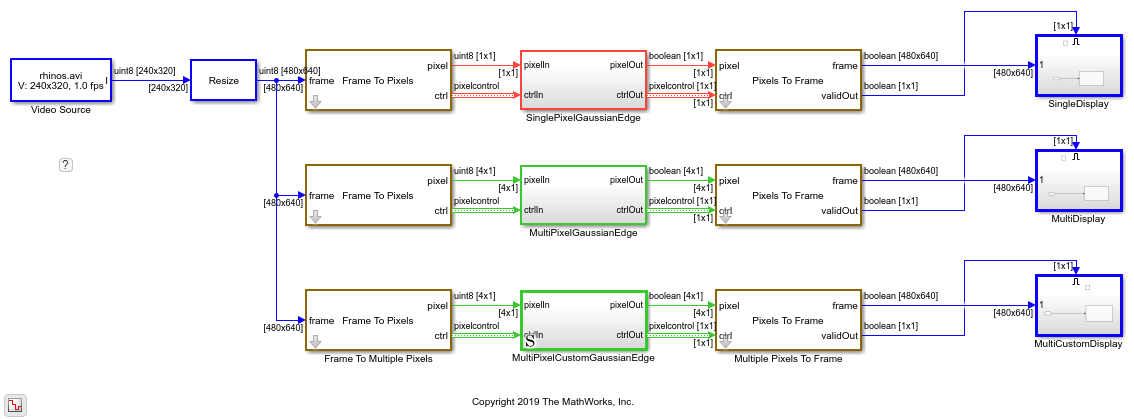### Multipixel Streaming Using Library Blocks

Generate a multipixel stream from the Frame to Pixels block by setting Number of pixels to `4` or `8`. The default value of `1` returns a scalar pixel stream with a sample rate of Total pixels per line * *Total video lines* faster than the frame rate. This rate shows red in the example model. The two multipixel subsystems use a multipixel stream with Number of pixels set to `4`. This configuration returns 4 pixels on each clock cycle and has a sample rate of (Total pixels per line/4) * *Total video lines*. The lower output rate, which is green in the model, shows that you can increase either the input frame rate or resolution by a factor of 4 and therefore process 4 times as many pixels in the same frame period using the same clock frequency as the single pixel case.

The SinglePixelGaussianEdge and MultiPixelGaussianEdge subsystems compute the same result using the Image Filter and Edge Detector blocks.

In MultiPixelGaussianEdge, the blocks accept and return four pixels on each clock cycle. You do not have to configure the blocks for multipixel streaming, they detect the input size on the port. The `pixelcontrol` bus indicates the validity and location in the frame of each set of four pixels. The blocks buffer the [4x1] stream to form four [ KernelHeight x KernelWidth ] kernels, and compute four convolutions in parallel to give a [4x1] output.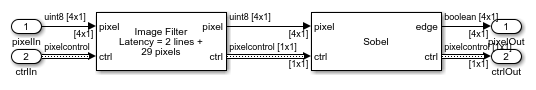### Custom Multipixel Algorithms

The MultiPixelCustomGaussianEdge subsystem uses the Line Buffer block to implement a custom filtering algorithm. This subsystem is similar to how the library blocks internally implement multipixel kernel operations. The Image Filter and Edge Detector blocks use more detailed optimizations than are shown here. This implementation shows a starting point for building custom multipixel algorithms using the output of the Line Buffer block.

The custom filter and custom edge detector use the Line Buffer block to return successive [ KernelHeight x NumberofPixels ] regions. Each region is passed to the KernelIndexer subsystem which uses buffering and indexing logic to form Number of Pixels * [ KernelHeight x KernelWidth ] filter kernels. Then each kernel is passed to a separate FilterKernel subsystem to perform convolutions in parallel.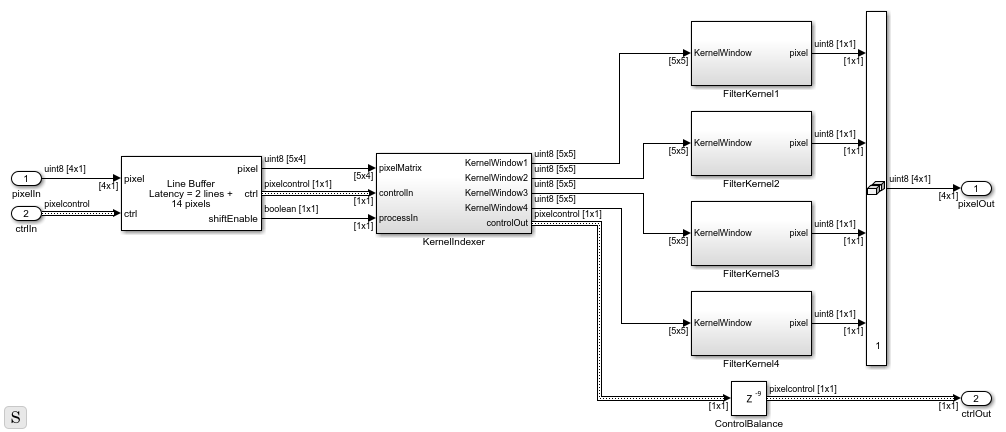### Form Kernels from Line Buffer Output

The KernelIndexer subsystem forms 4 [5x5] filter kernels from the 2-D output of the Line Buffer block.The diagram shows how the filter kernel is extracted from the [5x4] output stream, for the kernel that is centered on the first pixel in the [4x1] output. This first kernel includes pixels from 2 adjacent [5x4] Line Buffer outputs.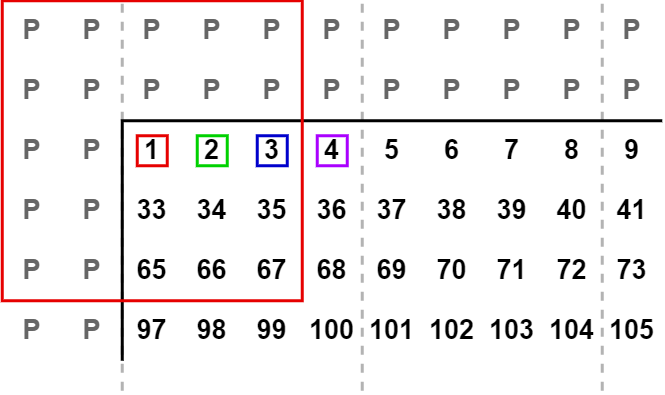The kernel centered on the last pixel in the [4x1] output also includes the third adjacent [5x4] output. So, to form four [5x5] kernels, the subsystem must access columns from three [5x4] matrices.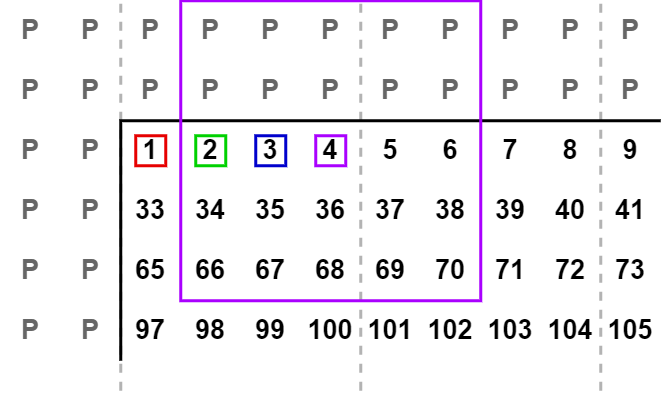The KernelIndexer subsystem uses the current [5x4] input, and stores two more [5x4] matrices using registers enabled by `shiftEnable`. This design is similar to the tapped delay line used with a Line Buffer using single pixel streaming. The subsystem then accesses pixel data across the columns to form the four [5x5] kernels. The Image Filter block uses this same logic internally when the block has multipixel input. The block automatically designs this logic at compile time for any supported kernel size.

### Implement Filters

Since the input multipixel stream is a [4x1] vector, the filters must perform four convolutions on each cycle to keep pace with the incoming data. There are four parallel FilterKernel subsystems that each perform the same operation. The [5x5] matrix multiply is implemented as a [5x1] vector multiply by using a For Each subsystem containing a pipelined multiplier. The output is passed to an adder tree. The adder tree is also pipelined, and the pipeline latency is applied to the `pixelcontrol` signal to match. The results of the four FilterKernel subsystems are then concatenated into a [4x1] output vector.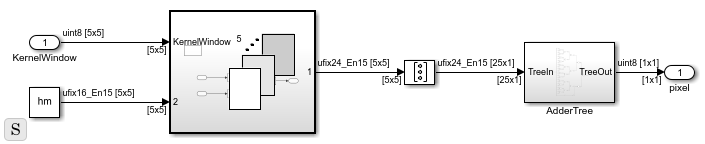### Implement Edge Detectors

To match the algorithm of the Edge Detector block, this custom edge detector uses a [3x3] kernel size. Compare this KernelIndexer subsystem for the [3x3] edge detection with the [5x5] kernel described above. The algorithm still must access three successive matrices from the output of the Line Buffer block (including padding on either side of the kernel). However, the algorithm saves fewer columns to form a smaller filter kernel.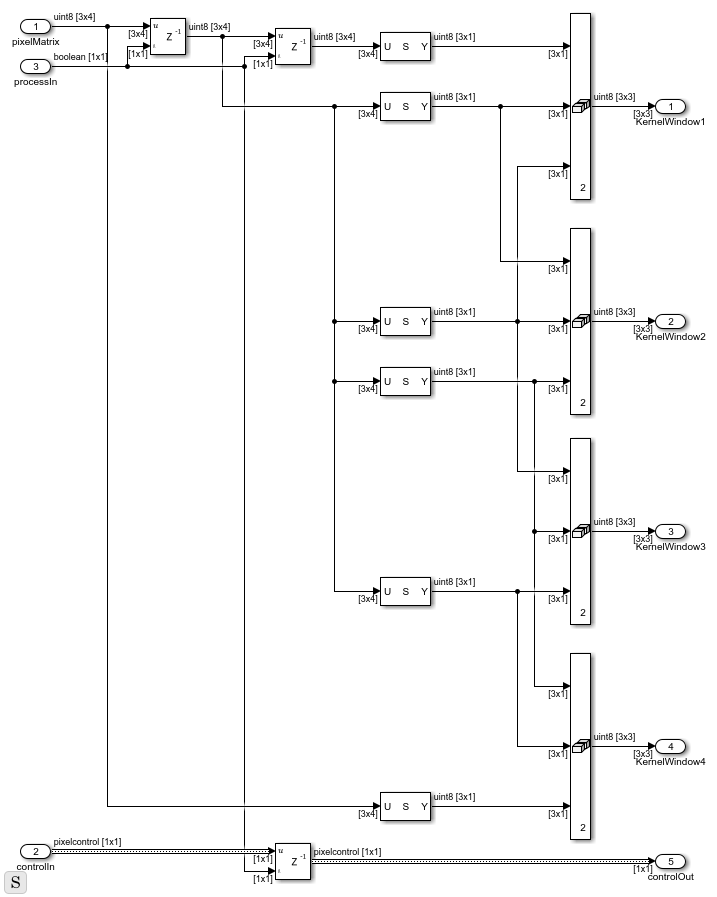### Extending to Larger Kernel Sizes

For larger kernel sizes the number of [ KernelHeight x NumPixels ] regions to store in the KernelIndexer is `(2 * ceil(floor(KernelWidth / 2) / NumPixels) + 1)`. In such a case, the number of inputs to the concatenators increases to KernelWidth and you must route these additional inputs from the tapped delay line of Line Buffer matrices. For a [4x1] multipixel stream with a [11x11] kernel size you would need to store five [11x4] matrices from the Line Buffer to form four [11x11] kernels each cycle.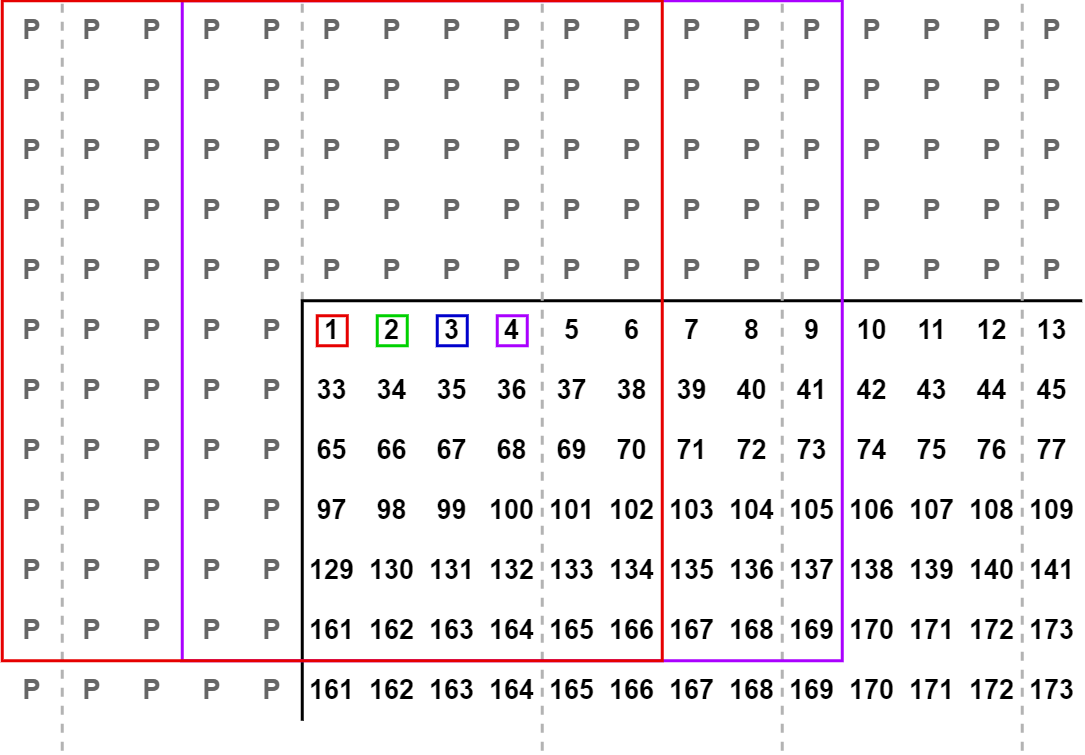### Improving Simulation Time

In the default example configuration, the single pixel, multipixel, and custom multipixel subsystems all run in parallel. The simulation speed is limited by the time processing the single-pixel path because it requires more iterations to process the same size of frame. To observe the simulation speed improvement for multipixel streaming, comment out the single-pixel data path.

### HDL Implementation Results

HDL was generated from both the MultiPixelGaussianEdge subsystem and the MultiPixelCustomGaussianEdge subsystem and put through Place and Route on a Xilinx™ ZC706 board. The MultiPixelCustomGaussianEdge subsystem, which does not attempt to optimize coefficients, had the following results -

```T = 4x2 table Resource Usage _________ _____ DSP48 108 Flip Flop 9842 LUT 4960 BRAM 12 ```

The MultiPixelGaussianEdge subsystem, which uses the optimized Image Filter and Edge Detector blocks uses less resources, as shown in the table below. This comparison shows the resource savings achieved because the blocks analyze the filter structure and pre-add repeated coefficients.

```T = 4x2 table Resource Usage _________ _____ DSP48 16 Flip Flop 3959 LUT 1789 BRAM 10 ```